## Exercise 3.1 Page No: 3.4

1. Write each of the following as decimals:
(i) (8/100)
(ii) 20 + (9/10) + (4/100)
(iii) 23 + (2/10) + (6/1000)
Solution:
(i) Given (8/100)
Mark the decimal point two places from right to left
(8/100) = 0.08
(ii) Given 20 + (9/10) + (4/100)
First convert the fractions (9/10) and (4/100) to decimals
Consider (9/10)
Mark the decimal point one place from right to left
(9/10) = 0.9
Now consider (4/100)
Mark the decimal point two places from right to left
(4/100) = 0.04
20 + (9/10) + (4/100) = 20 + 0.9 + 0.04
= 20.94
(iii) Given 23 + (2/10) + (6/1000)
First convert the fractions (2/10) and (6/1000) to decimals
Consider (2/10)
Mark the decimal point one place from right to left
(2/10) = 0.2
Now consider (6/1000)
Mark the decimal point three places from right to left
(6/1000) = 0.006
23 + (2/10) + (6/1000) = 23 + 0.2 + 0.006
= 23.206
2. Convert each of the following fractions as decimals:
(i) 0.04
(ii) 2.34
(iii) 0.342
(iv) 17.38
Solution:
(i) Given 0.04
Here we have to convert given decimals into fractions
0.04 can be written as (0.04/1)
Now multiply both numerator and denominator by 100 then we get
(0.04/1) = (0.04 × 100 /1 × 100)
= (4/100)
= (1/25)
(ii) Given 2.34
Here we have to convert given decimals into fractions
2.34 can be written as (2.34/1)
Now multiply both numerator and denominator by 100 then we get
(2.34/1) = (2.34 × 100 /1 × 100)
= (234/100)
= (117/50)
(iii) Given 0.342
Here we have to convert given decimals into fractions
0.342 can be written as (0.342/1)
Now multiply both numerator and denominator by 1000 then we get
(0.342/1) = (0.342 × 1000 /1 × 1000)
= (342/1000)
= (171/500)
(iv) Given 17.38
Here we have to convert given decimals into fractions
17.38 can be written as (17.38/1)
Now multiply both numerator and denominator by 100 then we get
(17.38/1) = (17.38 × 100 /1 × 100)
= (1738/100)
= (869/50)
3. Express the following fractions as decimals:
(i) (23/10)
(ii) 25 (1/8)
(iii) 39 (7/35)
(iv) 15 (1/25)
Solution:
(i) Given (23/10)
Divide 23 by 10 we get
(23/10) = 2.3
(ii) Given 25 (1/8)
25 (1/8) can be written as
25 (1/8) = 25 + (1/8)
Consider (1/8),
Now multiply both numerator and denominator by 125 to get 1000 as denominator
25 (1/8) = 25 + (1/8) = 25 + (1 × 125 /8 × 125)
= 25 + (125/1000)
= 25 + 0.125
= 25.125
(iii) Given 39 (7/35)
First convert given mixed fraction 39 (7/35) into improper fraction
39 (7/35) = 1372/35
By dividing we get
39 (7/35) = 39.2
(iv) Given 15 (1/25)
15 (1/25) can be written as
15 (1/25) = 15 + (1/25)
Consider (1/25),
Now multiply both numerator and denominator by 4 to get 100 as denominator
15 (1/25) = 15 + (1/25) = 15 + (1 × 4 /25 × 4)
= 15 + (4/100)
= 15 + 0.04
= 15.04
(i) 41.8, 39.24, 5.01 and 62.6
(ii) 18.03, 146.3, .829 and 5.324
Solution:
(i) Given 41.8, 39.24, 5.01 and 62.6
41.8
39.2
5.01
+ 62.6
148.65
(ii) Given 18.03, 146.3, 0.829 and 5.324
18.03
146.3
0.829
+ 5.324
170.483
5. Find the value of:
(i) 9.756 – 6.28
(ii) 48.1 – 0.37
(iii) 108.032 – 86.8
(iv) 100 – 26.32
Solution:
(i) Given 9.756 – 6.28
9.756
– 6.28
3.476
(ii) Given 48.1 – 0.37
48.1
– 0.37
47.73
(iii) Given 108.032 – 86.8
108.032
– 86.8
21.232
(iv) Given 100 – 26.32
100.00
– 26.32
73.68
6. Take out of 3.547 from 7.2

Solution:
Given 3.547 from 7.2
7.2
– 3.547
3.653
7. What is to be added to 36.85 to get 59.41?
Solution:
Given 36.85 and 59.41
Let the unknown number be x
x + 36.85 = 59.41
x = 59.41 – 36.85
x = 22.56
Hence 22.56 is to be added to 36.85 to get 59.41
8. What is to be subtracted from 17.1 to get 2.051?
Solution:
Let the unknown number be x
Given that x is to be subtracted from 17.1 to get 2.051
17.1 – x = 2.051
17.1 – 2.051 = x
x = 17.1 – 2.051
x = 15.049
9. By how much should 34.79 be increased to get 70.15?
Solution:
Let x be the unknown number
x + 34.79 = 70.15
x = 70.15 – 34.79
x = 35.36
35.36 should be increased to 34.79 to get 70.15
10. By how much should 59.71 be decreased to get 34.58?
Solution:
Let x be the unknown number
59.71 – x =34.58
59.71 – 34.58 = x
x = 59.71 – 34.58
x = 25.13
25.13 should be decreased by 59.71 to get 34.58

## Exercise 3.2 Page No: 3.8

1. Find the product:
(i) 4.74 × 10
(ii) 0.45 × 10
(iii) 0.0215 × 10
(iv) 0.0054 × 10
Solution:
(i) Given 4.74 × 10
Here we have to do normal multiplication with shifting the decimal point by one place to the right
Therefore 4.74 × 10 = 47.4
(ii) Given 0.45 × 10
Here we have to do normal multiplication with shifting the decimal point by one place to the right
Therefore 0.45 × 10 = 4.5
(iii) Given 0.0215 × 10
Here we have to do normal multiplication with shifting the decimal point by one place to the right
Therefore 0.0215 × 10 = 0.215
(iv) Given 0.0054 × 10
Here we have to do normal multiplication with shifting the decimal point by one place to the right
Therefore 0.0054 × 10 = 0.054
2. Find the product:
(i) 35.853 × 100
(ii) 42.5 × 100
(iii) 12.075 × 100
(iv) 100 × 0.005
Solution:
(i) Given 35.853 × 100
Here we have to do normal multiplication with shifting the decimal point by two places to the right
Therefore 35.853 × 100 = 3585.3
(ii) Given 42.5 × 100
Here we have to do normal multiplication with shifting the decimal point by two places to the right
Therefore 42.5 × 100 = 4250
(iii) Given 12.075 × 100
Here we have to do normal multiplication with shifting the decimal point by two places to the right
Therefore 12.075 × 100 = 1207.50
(iv) Given 100 × 0.005
Here we have to do normal multiplication with shifting the decimal point by two places to the right
Therefore 100 × 0.005 = 0.5
3. Find the product:
(i) 2.506 × 1000
(ii) 20.708 × 1000
(iii) 0.0529 × 1000
(iv) 1000 × 0.1
Solution:
(i) Given 2.506 × 1000
Here we have to do normal multiplication with shifting the decimal point by three places to the right
Therefore 2.506 × 1000 = 2506
(ii) Given 20.708 × 1000
Here we have to do normal multiplication with shifting the decimal point by three places to the right
Therefore 20.708 × 1000 = 20708
(iii) Given 0.0529 × 1000
Here we have to do normal multiplication with shifting the decimal point by three places to the right
Therefore 0.0529 × 1000 = 52.9
(iv) Given 1000 × 0.1
Here we have to do normal multiplication with shifting the decimal point by three places to the right
Therefore 1000 × 0.1 = 100
4. Find the product:
(i) 3.14 × 17
(ii) 0.745 × 12
(iii) 28.73 × 47
(iv) 0.0415 × 59
Solution:
(i) Given 3.14 × 17
First multiply as usual without looking at the decimal point
3.14 × 17 = 578
Now mark the decimal point in the product to have one place of decimal as there in the given decimal
3.14 × 17 = 57.8
(ii) Given 0.745 × 12
First multiply as usual without looking at the decimal point
0.745 × 12 = 894
Now mark the decimal point in the product to have three places of decimal as there in the given decimal
0.745 × 12 = 8.94
(iii) Given 28.73 × 47
First multiply as usual without looking at the decimal point
28.73 × 47 = 135031
Now mark the decimal point in the product to have two places of decimal as there in the given decimal
28.73 × 47 = 1350.31
(iv) Given 0.0415 × 59
First multiply as usual without looking at the decimal point
0.0415 × 59 = 24485
Now mark the decimal point in the product to have four places of decimal as there in the given decimal
0.0415 × 59 = 2.4485
5. Find:
(i) 1.07 × 0.02
(ii) 211.9 × 1.13
(iii) 10.05 × 1.05
(iv) 13.01 × 5.01
Solution:
(i) Given 1.07 × 0.02
First multiply as usual without looking at the decimal point
1.07 × 0.02 = 00214
Sum of the decimals is 4
Now mark the decimal point in the product to have four places of decimal as there in the given decimal
1.07 × 0.02 = 0.0214
(ii) Given 211.9 × 1.13
First multiply as usual without looking at the decimal point
211.9 × 1.13 = 239447
Sum of the decimals is 3
Now mark the decimal point in the product to have three places of decimal as there in the given decimal
211.9 × 1.13 = 239.447
(iii) Given 10.05 × 1.05
First multiply as usual without looking at the decimal point
10.05 × 1.05 = 105525
Sum of the decimals is 4
Now mark the decimal point in the product to have four places of decimal as there in the given decimal
10.05 × 1.05 = 10.5525
(iv) Given 13.01 × 5.01
First multiply as usual without looking at the decimal point
13.01 × 5.01 = 651801
Sum of the decimals is 4
Now mark the decimal point in the product to have four places of decimal as there in the given decimal
13.01 × 5.01 = 65.1801
6. Find the area of a rectangle whose length is 5.5m and breadth is 3.4m.
Solution:
Given length of rectangle = 5.5m
Breadth of rectangle = 3.4 m
Area of rectangle = length × breadth
= 5.5 × 3.4
= 18.7 m2
7. If the cost of a book is Rs 25.57, find the cost 0f 24 such books.
Solution:
Given cost of a book is Rs 25.57
Cost of 24 books = 25.57 × 24
= Rs 618.00
8. A car covers a distance of 14.75km in one liter of petrol. How much distance it will cover in 15.5 liters of petrol?
Solution:
Given that distance covered by car in 1 liter of petrol = 14.75 km
Distance covered by car in 15.5 liters of petrol = 14.75 × 15.5
= 228.625 km
9. One kg of rice costs Rs 42.65. What will be the cost of 18.25 kg of rice?
Solution:
Given cost of 1kg of rice = 42.65
Cost of 18.25kg of rice = 42.65 × 18.25
= Rs 778.3625
10. One meter of cloth costs Rs 152.50. What is the cost of 10.75 meters of cloth?
Solution:
Given that cost of 1m cloth = Rs 152.50
Cost of 10.75 m of cloth = 152.50 × 10.75
= Rs 1639.375

## Exercise 3.3 Page No: 3.16

1. Divide:
(i) 142.45 by 10
(ii) 54.25 by 10
(iii) 3.45 by 10
(iv) 0.57 by 10
(v) 0.0043 by 10
(vi) 0.004 by 10
Solution:
(i) Given 142.45 by 10
Now shifting the decimal point by one place to the left we can get the result
142.45/10 = 14.245
(ii) Given 54.25 by 10
Now shifting the decimal point by one place to the left we can get the result
54.25/10 = 5.425
(iii) Given 3.45 by 10
Now shifting the decimal point by one place to the left we can get the result
3.45/10 = 0.345
(iv) Given 0.57 by 10
Now shifting the decimal point by one place to the left we can get the result
0.57/10 = 0.057
(v) Given 0.0043 by 10
Now shifting the decimal point by one place to the left we can get the result
0.0043/10 = 0.0043
(vi) Given 0.004 by 10
Now shifting the decimal point by one place to the left we can get the result
0.004/10 = 0.0004
2. Divide:
(i) 459.5 by 100
(ii) 74.3 by 100
(iii) 5.8 by 100
(iv) 0.7 by 100
(v) 0.48 by 100
(vi) 0.03 by 100
Solution:
(i) Given 459.5 by 100
Now shifting the decimal point by two places to the left we can get the result
459.5/100 = 4.595
(ii) Given 74.3 by 100
Now shifting the decimal point by two places to the left we can get the result
74.3/100 = 0.743
(iii) Given 5.8 by 100
Now shifting the decimal point by two places to the left we can get the result
5.8/100 = 0.058
(iv) Given 0.7 by 100
Now shifting the decimal point by two places to the left we can get the result
0.7/100 = 0.007
(v) Given 0.48 by 100
Now shifting the decimal point by two places to the left we can get the result
0.48/100 = 0.0048
(vi) Given 0.03 by 100
Now shifting the decimal point by two places to the left we can get the result
0.03 / 100 = 0.0003
3. Divide:
(i) 235. 41 by 1000
(ii) 29.5 by 1000
(iii) 3.8 by 1000
(iv) 0.7 by 1000
Solution:
(i) Given 235. 41 by 1000
Now shifting the decimal point by three places to the left we can get the result
235. 41/1000 = 0.23541
(ii) Given 29.5 by 1000
Now shifting the decimal point by three places to the left we can get the result
29.5/1000 = 0.0295
(iii) Given 3.8 by 1000
Now shifting the decimal point by three places to the left we can get the result
3.8 /1000 = 0.0038
(iv) Given 0.7 by 1000
Now shifting the decimal point by three places to the left we can get the result
0.7 /1000 = 0.0007
4. Divide:
(i) 0.45 by 9
(ii) 217.44 by 18
(iii) 319.2 by 2.28
(iv) 40.32 by 9.6
(v) 0.765 by 0.9
(vi) 0.768 by 1.6
Solution:
(i) Given 0.45 by 9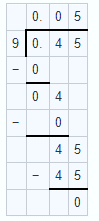0.45 by 9 = 0.05
(ii) Given 217.44 by 18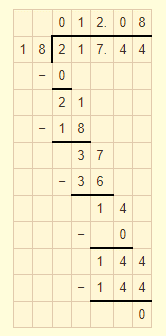217.44 by 18 = 12.08
3. Given 319.2 by 2.28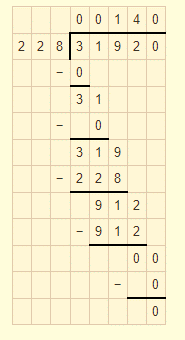319.2 by 2.28 = 140
(iv) Given 40.32 by 9.6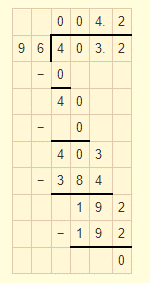40.32 by 9.6 = 4.2
(v) Given 0.765 by 0.9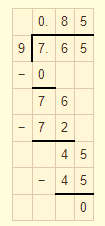0.765 by 0.9 = 0.85
(vi) Given 0.768 by 1.6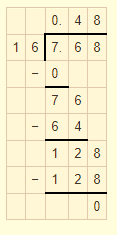0.768 by 1.6 = 0.48
5. Divide:
(i) 16.64 by 20
(ii) 0.192 by 12
(iii) 163.44 by 24
(iv) 403.2 by 96
(v) 16.344 by 12
(vi) 31.92 by 228
Solution:
(i) Given 16.64 by 20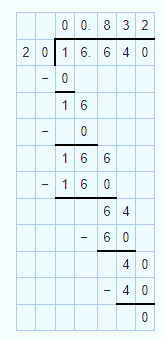16.64 by 20 = 0.832
(ii) Given 0.192 by 12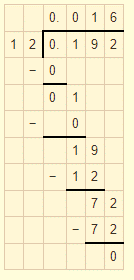0.192 by 12 = 0.016
(iii) Given 163.44 by 24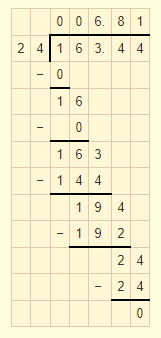163.44 by 24 = 6.81
(iv) Given 403.2 by 96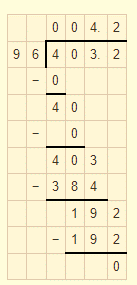403.2 by 96 = 4.2
(v) Given 16.344 by 12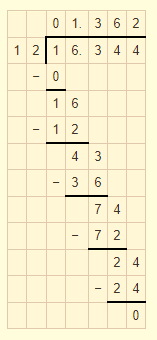16.344 by 12 = 1.362
(vi) Given 31.92 by 228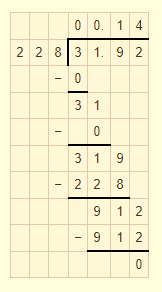31.92 by 228 = 0.14
6. Divide:
(i) 15.68 by 20
(ii) 164.6 by 200
(iii) 403.80 by 30
Solution:
(i) Given 15.68 by 20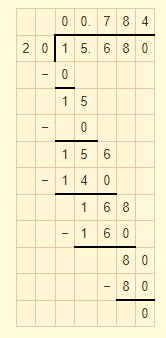15.68 by 20 = 0.784
(ii) Given 164.6 by 200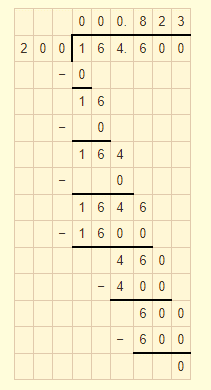164.6 by 200 = 0.823
(iii) Given 403.80 by 30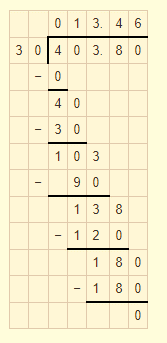403.80 by 30 = 13.46
7. Divide:
(i) 76 by 0.019
(ii) 88 by 0.08
(iii) 148 by 0.074
(iv) 7 by 0.014
Solution:
(i) Given 76 by 0.019
Multiply both numerator and denominator by 1000 then divide
= 76000/19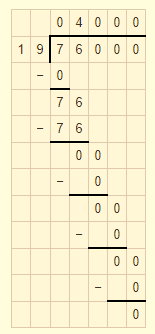76 by 0.019 = 4000
(ii) Given 88 by 0.08
Multiply both numerator and denominator by 100 then divide
= 8800/8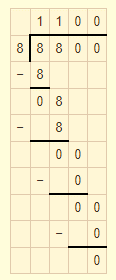88 by 0.08 = 1100
(iii) Given 148 by 0.074
Multiply both numerator and denominator by 1000 then divide
= 14800/74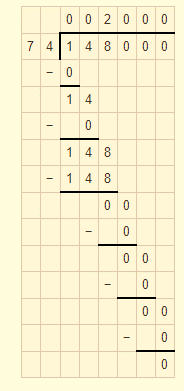148 by 0.074 = 2000
(iv) Given 7 by 0.014
Multiply both numerator and denominator by 1000 then divide
= 7000/14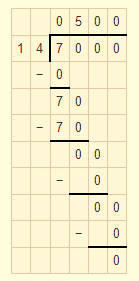7 by 0.014 = 500
8. Divide:
(i) 20 by 50
(ii) 8 by 100
(iii) 72 by 576
(iv) 144 by 15
Solution:
(i) Given 20 by 50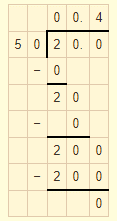20 by 50 = 0.4
(ii) Given 8 by 100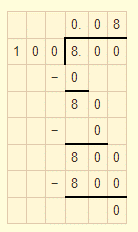8 by 100 = 0.08
(iii) Given 72 by 576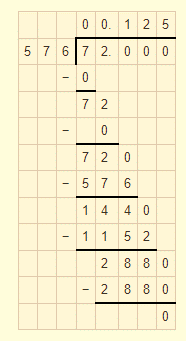72 by 576 = 0.125
(iv) Given 144 by 15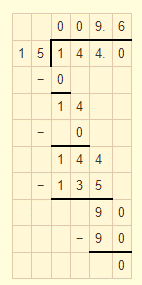144 by 15 = 9.6
9. A vehicle covers a distance 0f 43.2 km in 2.4 litres of petrol. How much distance will travel in 1 litre of petrol?
Solution:
Given distance covered by vehicle in 2.4 litres of petrol is = 43.2 km
Distance travel by vehicle in 1 litre of petrol = 43.2/2.4
Multiply both numerator and denominator by 10 then we get 432/24
On dividing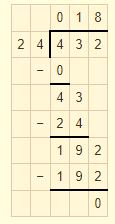Therefore 18km can travel in 1litre of petrol.
10. The total weight of some bags of wheat is 1743 kg. If each bag weighs 49.8 kg, how many bags are there?
Solution:
Given total weight of total bags = 1743 kg
Each bag weighs = 49.8 kg
Number of bags = 1743/49.8
Multiply both numerator and denominator by 10 then we get 17430/498
On dividing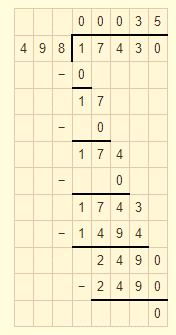Therefore number of bags are 35
11. Shikha cuts 50 m of cloth into pieces 0f 1.25 m each. How many pieces does she get?
Solution:
Given that total length of cloth = 50 m
Length of each piece = 1.25 m
Number of cloth piece = 50/1.25
Multiply both numerator and denominator by 100 then we get 5000/125
On dividing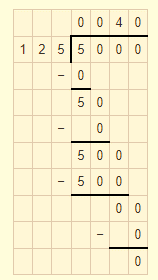Number of cloth piece = 40
12. Each side of a rectangular polygon is 2.5cm in length. The perimeter of the polygon is 12.5 cm. How many sides does the polygon have?
Solution:
Given that length of each side of polygon is = 2.5 cm
Perimeter of polygon = 12.5 cm
Number of sides = 12.5/2.5
Multiply both numerator and denominator by 10 then we get 125/25
On dividing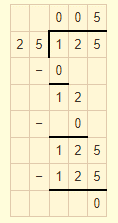Number of sides of polygon is 5
13. The product of two decimals is 42.987. If one of them is 12.46, find the other.
Solution:
Given that product of two decimals = 42.987
One of the number is = 12.46
Another number is = 42.987/12.46
Multiply both numerator and denominator by 1000 then we get 42987/12460
On dividing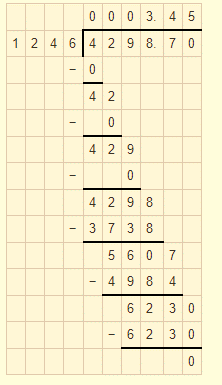Another number is 3.45
14. The weight of 34 bags of sugar is 3483.3 kg. If all bags weigh equally, find the weight of each bag.
Solution:
Given that weight of 34 bags of sugar is = 3483.3 kg
Weight of each bag = 3483.3/34
Multiply both numerator and denominator by 10 then we get 34833/34
On dividing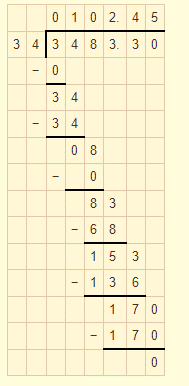Weight of each bag = 1o2.45 kg
15. How many buckets of equal capacity can be filled from 586.5 litres of water, if each has capacity of 8.5 litres?
Solution:
Given that capacity of each bucket = 8.5 litres
Total water available = 586.5 litres
Number of buckets = 586.5/8.5
Multiply both numerator and denominator by 10 then we get 5865/85
On dividing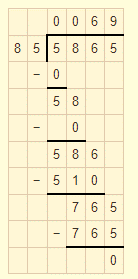Number of buckets = 69
Courtesy : CBSE